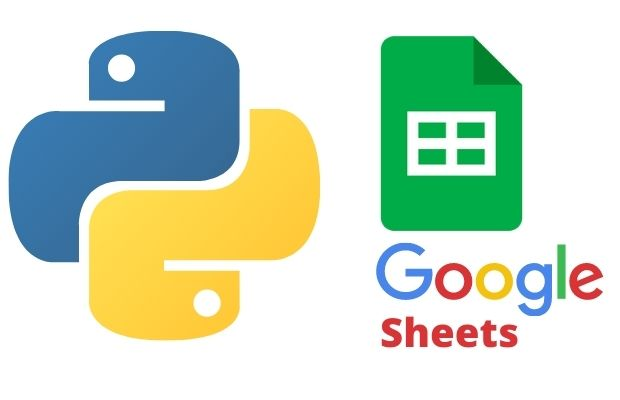# Pandas DataFrame & Google sheetsCreate one folder inside your google drive, give the name as python-test.
Give Share authorization ( Share with people and group ) to Google API service account you are using.

Creating one new File my_sheet1 ( google sheet ) inside python-test directory.
``````import pygsheets
gc=pygsheets.authorize(service_account_file=path)
sh=gc.create('my_sheet1',folder_name='python-test')``````

## set_dataframe

``````set_dataframe(df, start, copy_index=False, copy_head=True,
extend=False, fit=False, escape_formulae=False, **kwargs)``````
OptionsDetails
dfPandas DataFrame
startStaring Point tuple ( row, column). It is (1,1) in below example
copy_indexDefault False, Copy Index data from DataFrame
extendFalse( default) If required add columns and rows ( see example )
fitFalse(default), Resize to fit all data in DataFrame if required
escape_formulaeHow to handle formula ( value with = or +- sign )
nanReplace NaN values with
Create Pandas DataFrame and then store the same inside our google sheets my_sheet1.
``````wk1=sh # first worksheet
import pandas as pd  # importing Pandas library
my_dict={
'NAME':['Ravi','Raju','Alex'],
'ID':[1,2,3],'MATH':[30,40,50],
'ENGLISH':[20,30,40]
}
df = pd.DataFrame(data=my_dict) # creating DataFrame
wk1.set_dataframe(df,(1,1))``````

Subscribe to our YouTube Channel here

## Subscribe

* indicates required
Subscribe to plus2netplus2net.com